### Practice Set 3.3 Arithmetic Progression Class 10th Mathematics Part 1 MHB Solution

Practice Set 3.3

1. First term and common difference of an A.P. are 6 and 3 respectively ; find S27. a = 6,…
2. Find the sum of first 123 even natural numbers.
3. Find the sum of all even numbers from 1 to 350.
4. In an A.P. 19th term is 52 and 38th term is 128, find sum of first 56 terms.…
5. Complete the following activity to find the sum of natural numbers from 1 to 140 which…
6. Sum of first 55 terms in an A.P. is 3300, find its 28th term.
7. In an A.P. sum of three consecutive terms is 27 and their product is 504 find the…
8. Find four consecutive terms in an A.P. whose sum is 12 and sum of 3rd and 4th term is…
9. If the 9th term of an A.P. is zero then show that the 29th term is twice the 19th term.…

###### Practice Set 3.3

Question 1.

First term and common difference of an A.P. are 6 and 3 respectively ; find S27.

a = 6, d = 3, S27 = ?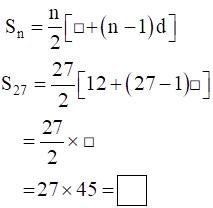Given: First term a = 6

Common Difference d = 3

To find: S27 where n = 27

By using sum of nth term of an A.P. is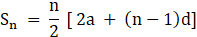Where, n = no. of terms

a = first term

d = common difference

Sn = sum of n terms

Thus, Substituting given value in formula we can find the value of S27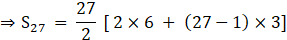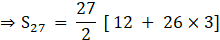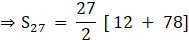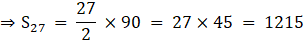Thus, S27 = 1215

Question 2.

Find the sum of first 123 even natural numbers.

List of first 123 even natural number is

2,4,6,…….

Where first term a = 2

Second term t1 = 4

Third term t2 = 6

Thus, common difference d = t2 – t1 = 6 – 4 = 2

n = 123

By using sum of nth term of an A.P. isWhere, n = no. of terms

a = first term

d = common difference

Sn = sum of n terms

Thus, Substituting given value in formula we can find the value of Sn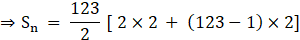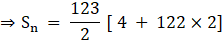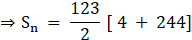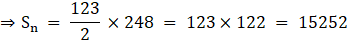Thus, Sn = 15252

Question 3.

Find the sum of all even numbers from 1 to 350.

List of even natural number between 1 to 350 is

2,4,6,…….348

Where first term a = 2

Second term t1 = 4

Third term t2 = 6

Thus, common difference d = t2 – t1 = 6 – 4 = 2

tn = 348 (As we have to find the sum of even numbers between 1 and 350 therefore excluding 350 )

Now, By using nth term of an A.P. formula

tn = a + (n – 1)d

where n = no. of terms

a = first term

d = common difference

tn = nth terms

we can find value of “n” by substituting all the value in formula we get,

⇒ 348 = 2 + (n – 1) × 2

⇒ 348 – 2 = 2(n – 1)

⇒ 346 = 2(n – 1)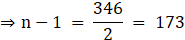⇒ n = 173 + 1 = 174

Now, By using sum of nth term of an A.P. we will find it’s sumWhere, n = no. of terms

a = first term

d = common difference

Sn = sum of n terms

Thus, Substituting given value in formula we can find the value of Sn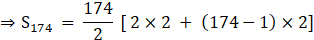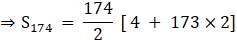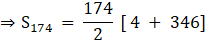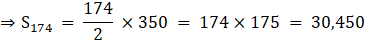Thus, S174 = 30,450

Question 4.

In an A.P. 19th term is 52 and 38th term is 128, find sum of first 56 terms.

Given: t19 = 52 and t38 = 128

To find: value of “a” and “d”

Using nth term of an A.P. formula

tn = a + (n – 1)d

where n = no. of terms

a = first term

d = common difference

tn = nth terms

we will find value of “a” and “d”

Let, t19 = a + (19 – 1) d

⇒ 52 = a + 18 d …..(1)

t38 = a + (38 – 1) d

⇒ 128 = a + 37 d …..(2)

Subtracting eq. (1) from eq. (2), we get,

⇒ 128 – 52 = (a – a) + (37 d – 18 d)

⇒ 76 = 19 d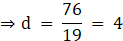Substitute value of “d” in eq. (1) to get value of “a”

⇒ 52 = a + 18 ×4

⇒ 52 = a + 72

⇒ a = 52 – 72 = – 20

Now, to find value of S56 we will using formula of sum of n termsWhere, n = no. of terms

a = first term

d = common difference

Sn = sum of n terms

Thus, Substituting given value in formula we can find the value of Sn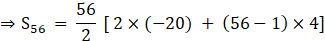⇒S56 = 28 × [ – 40 + 55×4]

⇒S56 = 28 × [ – 40 + 220]

⇒S56 = 28 × 180 = 5040

Thus, S56 = 5040

Question 5.

Complete the following activity to find the sum of natural numbers from 1 to 140 which are divisible by 4.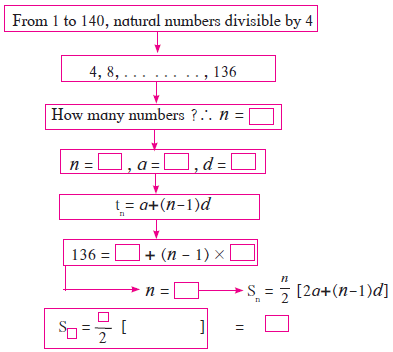Sum of numbers from 1 to 140, which are divisible by 4 =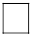List of natural number divisible by 4 between 1 to 140 is

4,8,12,…….136

Where first term a = 4

Second term t1 = 8

Third term t2 = 12

Thus, common difference d = t2 – t1 = 12 – 8 = 4

tn = 136

Now, By using nth term of an A.P. formula

tn = a + (n – 1)d

where n = no. of terms

a = first term

d = common difference

tn = nth terms

we can find value of “n” by substituting all the value in formula we get,

⇒ 136 = 4 + (n – 1) × 4

⇒ 136 – 4 = 4(n – 1)

⇒ 132 = 4(n – 1)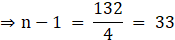⇒ n = 33 + 1 = 34

Now, By using sum of nth term of an A.P. we will find it’s sumWhere, n = no. of terms

a = first term

d = common difference

Sn = sum of n terms

Thus, Substituting given value in formula we can find the value of S34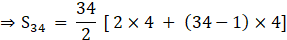⇒S34 = 17 × [8 + 33×4]

⇒S34 = 17 × [8 + 132]

⇒S34 = 17 × 140 = 2380

Thus, S34 = 2380

Question 6.

Sum of first 55 terms in an A.P. is 3300, find its 28th term.

Given: S55 = 3300 where n = 55

Now, By using sum of nth term of an A.P. we will find it’s sumWhere, n = no. of terms

a = first term

d = common difference

Sn = sum of n terms

Thus, on substituting the given value in formula we get,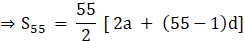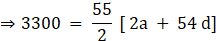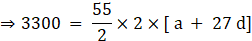⇒ 3300 = 55 × [ a + 27d]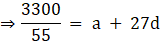⇒ a + 27d = 60 ……(1)

We need to find value of 28th term i.e t28

Now, By using nth term of an A.P. formula

tn = a + (n – 1)d

where n = no. of terms

a = first term

d = common difference

tn = nth terms

we can find value of t28 by substituting all the value in formula we get,

⇒ t28 = a + (28 – 1) d

⇒ t28 = a + 27 d

From eq. (1) we get,

⇒ t28 = a + 27 d = 60

⇒ t28 = 60

Question 7.

In an A.P. sum of three consecutive terms is 27 and their product is 504 find the terms? (Assume that three consecutive terms in A.P. are a – d, a, a + d.)

Let the first term be a – d

the second term be a

the third term be a + d

Given: sum of consecutive three term is 27

⇒ (a – d) + a + (a + d) = 27

⇒ 3 a = 27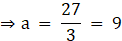Also, Given product of three consecutive term is 504

⇒ (a – d)× a × (a + d) = 504

⇒ (9 – d) × 9 × (9 + d) = 504 (since, a = 9)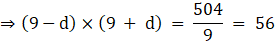⇒ 92 – d2 = 56 (since, (a – b)(a + b) = a2 – b2)

⇒ 81 – d2 = 56

⇒ d2 = 81 – 56 = 25

⇒ d = √25 = ± 5

Case 1:

Thus, if a = 9 and d = 5

Then the three terms are,

First term a – d = 9 – 5 = 4

Second term a = 9

Third term a + d = 9 + 5 = 14

Thus, the A.P. is 4, 9, 14

Case 2:

Thus, if a = 9 and d = – 5

Then the three terms are,

First term a – d = 9 – ( – 5) = 9 + 5 = 14

Second term a = 9

Third term a + d = 9 + ( – 5) = 9 – 5 = 4

Thus, the A.P. is 14, 9, 4

Question 8.

Find four consecutive terms in an A.P. whose sum is 12 and sum of 3rd and 4th term is 14.

(Assume the four consecutive terms in A.P. are a – d, a, a + d, a + 2d.)

Let the first term be a – d

the second term be a

the third term be a + d

the fourth term be a + 2 d

Given: sum of consecutive four term is 12

⇒ (a – d) + a + (a + d) + (a + 2d) = 12

⇒ 4 a + 2d = 12

⇒ 2(2 a + d) = 12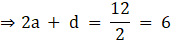⇒ 2a + d = 6 …..(1)

Also, sum of third and fourth term is 14

⇒ (a + d) + (a + 2d) = 14

⇒ 2a + 3d = 14 ……(2)

Subtracting eq. (1) from eq. (2) we get,

⇒(2a + 3d) – (2a + d) = 14 – 6

⇒2a + 3d – 2a – d = 8

⇒ 2d = 8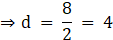⇒ d = 4

Substituting value of “d” in eq. (1) we get,

⇒ 2a + 4 = 6

⇒ 2a = 6 – 4 = 2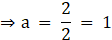⇒ a = 1

Thus, a = 1 and d = 4

Hence, first term a – d = 1 – 4 = – 3

the second term a = 1

the third term a + d = 1 + 4 = 5

the fourth term a + 2 d = 1 + 2×4 = 1 + 8 = 9

Thus, the A.P. is – 3, 1, 5, 9

Question 9.

If the 9th term of an A.P. is zero then show that the 29th term is twice the 19th term.

Now, By using nth term of an A.P. formula

tn = a + (n – 1)d

where n = no. of terms

a = first term

d = common difference

tn = nth terms

Given: t9 = 0

⇒ t9 = a + (9 – 1)d

⇒ 0 = a + 8d

⇒ a = – 8d

To Show: t29 = 2× t19

Now,

⇒ t29 = a + (29 – 1)d

⇒ t29 = a + 28d

⇒ t29 = – 8d + 28d = 20 d (since, a = – 8d )

⇒ t29 = 20 d

⇒ t29 = 2 × 10 d ….(1)

Also,

⇒ t19 = a + (19 – 1)d

⇒ t19 = a + 18d

⇒ t19 = – 8d + 18d = 10 d (since, a = – 8d )

⇒ t19 = 10 d …..(2)

From eq. (1) and eq. (2) we get,

t29 = 2× t19

## PDF FILE TO YOUR EMAIL IMMEDIATELY PURCHASE NOTES & PAPER SOLUTION. @ Rs. 50/- each (GST extra)

SUBJECTS

HINDI ENTIRE PAPER SOLUTION

MARATHI PAPER SOLUTION
SSC MATHS I PAPER SOLUTION
SSC MATHS II PAPER SOLUTION
SSC SCIENCE I PAPER SOLUTION
SSC SCIENCE II PAPER SOLUTION
SSC ENGLISH PAPER SOLUTION
SSC & HSC ENGLISH WRITING SKILL
HSC ACCOUNTS NOTES
HSC OCM NOTES
HSC ECONOMICS NOTES
HSC SECRETARIAL PRACTICE NOTES

2019 Board Paper Solution

HSC ENGLISH SET A 2019 21st February, 2019

HSC ENGLISH SET B 2019 21st February, 2019

HSC ENGLISH SET C 2019 21st February, 2019

HSC ENGLISH SET D 2019 21st February, 2019

SECRETARIAL PRACTICE (S.P) 2019 25th February, 2019

HSC XII PHYSICS 2019 25th February, 2019

CHEMISTRY XII HSC SOLUTION 27th, February, 2019

OCM PAPER SOLUTION 2019 27th, February, 2019

HSC MATHS PAPER SOLUTION COMMERCE, 2nd March, 2019

HSC MATHS PAPER SOLUTION SCIENCE 2nd, March, 2019

SSC ENGLISH STD 10 5TH MARCH, 2019.

HSC XII ACCOUNTS 2019 6th March, 2019

HSC XII BIOLOGY 2019 6TH March, 2019

HSC XII ECONOMICS 9Th March 2019

SSC Maths I March 2019 Solution 10th Standard11th, March, 2019

SSC MATHS II MARCH 2019 SOLUTION 10TH STD.13th March, 2019

SSC SCIENCE I MARCH 2019 SOLUTION 10TH STD. 15th March, 2019.

SSC SCIENCE II MARCH 2019 SOLUTION 10TH STD. 18th March, 2019.

SSC SOCIAL SCIENCE I MARCH 2019 SOLUTION20th March, 2019

SSC SOCIAL SCIENCE II MARCH 2019 SOLUTION, 22nd March, 2019

XII CBSE - BOARD - MARCH - 2019 ENGLISH - QP + SOLUTIONS, 2nd March, 2019

HSC Maharashtra Board Papers 2020

(Std 12th English Medium)

HSC ECONOMICS MARCH 2020

HSC OCM MARCH 2020

HSC ACCOUNTS MARCH 2020

HSC S.P. MARCH 2020

HSC ENGLISH MARCH 2020

HSC HINDI MARCH 2020

HSC MARATHI MARCH 2020

HSC MATHS MARCH 2020

SSC Maharashtra Board Papers 2020

(Std 10th English Medium)

English MARCH 2020

HindI MARCH 2020

Hindi (Composite) MARCH 2020

Marathi MARCH 2020

Mathematics (Paper 1) MARCH 2020

Mathematics (Paper 2) MARCH 2020

Sanskrit MARCH 2020

Important-formula

THANKS